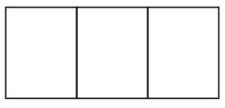Q

# Here is an incomplete net for making a cube. Complete it in at least two different ways. Remember that a cube has six faces. How many are there in the net here?(Give two separate diagrams. If you like, you may use a squared sheet for easy manipulation.)

Q 4    Here is an incomplete net for making a cube. Complete it in at least two different
ways. Remember that a cube has six faces. How many are there in the net here?
(Give two separate diagrams. If you like, you may use a squared sheet for easy
manipulation.)